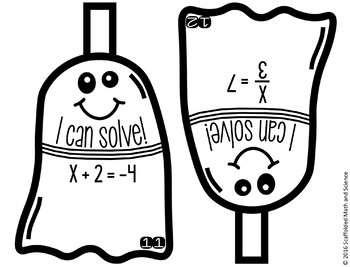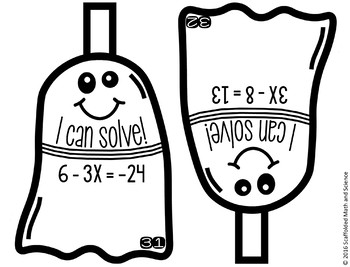Halloween Solving Equations Math Pennants6th - 8th
Subjects
Standards
Resource Type
Formats Included
• PDF
Pages
25 pages

Also included in

1. Students can practice their math while decorating your classroom for Halloween and Thanksgiving. In this bundle are 4 math pennant activities that cover solving proportions, solving equations, 2-digit multiplication and rounding decimals. The finished pennants make a colorful fall display and a crea
\$10.00
\$17.00
Save \$7.00
2. This bundle currently includes 38 algebra pennants and will be updated with additional sets when they are created. You will be notified when a new set is added and will be able to re-download to get the new sets free of charge. Math pennants are a fun way to show off student work, create colorful bu
\$70.00
\$127.00
Save \$57.00
3. This bundle contains 29 selected middle school math pennants. “This was one of the things that many of my students commented that they enjoyed when I asked them at the end of the year. They liked the opportunity to be somewhat creative. I think they felt like they were less threatening than "real" w
\$50.00
\$90.50
Save \$40.50

Description

Students solve 1-step and 2-step equations in this spooky math activity that doubles as a fun display of student work. Equations have variables on the left or the right and include negative numbers.

Included are 40 ghost pennants, optional student answer sheets and answer keys. 20 of the pennants cover 1-step equations and 20 cover 2-step equations.

Once a pennant is complete, it can be hung along a string in your classroom to show the world that, "Hey, we know how to solve equations!" Students can also choose to color their ghosts, which creates a festive classroom display.

Each group of students can be given a set of pennants, scissors and either glue, tape or a stapler. Students can be in charge of cutting out the pennants. Groups can compete to see which group can complete the most pennants or your entire class can add pennants to one string as they complete each one. You may also choose to have your students color their pennants to add to your classroom décor.

See all holiday math activities.

You may also like:

1-Step Equations Digital Math Escape Room

2-Step Equations Digital Math Escape Room

Graphing Linear Equations Ornaments

8th Grade Math Word Wall - print and digital

Total Pages
25 pages
Included
Teaching Duration
N/A
Report this Resource to TpT
Reported resources will be reviewed by our team. Report this resource to let us know if this resource violates TpT’s content guidelines.

Standards

to see state-specific standards (only available in the US).
Solve linear equations in one variable.
Use variables to represent quantities in a real-world or mathematical problem, and construct simple equations and inequalities to solve problems by reasoning about the quantities.
Solve multi-step real-life and mathematical problems posed with positive and negative rational numbers in any form (whole numbers, fractions, and decimals), using tools strategically. Apply properties of operations to calculate with numbers in any form; convert between forms as appropriate; and assess the reasonableness of answers using mental computation and estimation strategies. For example: If a woman making \$25 an hour gets a 10% raise, she will make an additional 1/10 of her salary an hour, or \$2.50, for a new salary of \$27.50. If you want to place a towel bar 9 3/4 inches long in the center of a door that is 27 1/2 inches wide, you will need to place the bar about 9 inches from each edge; this estimate can be used as a check on the exact computation.
Solve real-world and mathematical problems by writing and solving equations of the form 𝘹 + 𝘱 = 𝘲 and 𝘱𝘹 = 𝘲 for cases in which 𝘱, 𝘲 and 𝘹 are all nonnegative rational numbers.
Understand solving an equation or inequality as a process of answering a question: which values from a specified set, if any, make the equation or inequality true? Use substitution to determine whether a given number in a specified set makes an equation or inequality true.